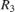# Hardware-Efficient Euler Rotations Using CORDIC

This example shows how to implement Euler rotations using a CORDIC kernel. The resulting model is deployable to FPGA or ASIC devices.

### Euler Rotations

Euler rotations are a common convention for describing arbitrary three dimensional rotations. You can modify them to describe either rotation of a vector in a fixed coordinate system, or rotation of a coordinate system with respect to a fixed vector. In this example, assume rotations of vectors with respect to fixed coordinate systems.

You can perform an Euler rotation of a vector as follows. First, rotate the vector byabout the z-axis. Then, rotate byabout the resultant x-axis. Finally, rotate byabout the rotated z-axis to obtain the final coordinates of the vector.

It is common to construct Euler rotations by multiplying three individual rotation matrices. For example, you can rotate a vectorusing the matrix transformation below.The matrixrepresents a rotation about the axis. For example, the matrixis given byWhile this form is easily understood, it has several inefficiencies. If,, andare variables, you must recalculateandfor each angle prior to forming up the matrix. You further need to multiply and add these results, which can result in unnecessary word length growth. Finally, the entire matrix multiplication must be properly pipelined for maximum efficiency. This can be a time consuming process.

### Deploy Euler Rotations Using CORDIC Algorithm

Euler rotations operate by performing rotations in planes of intersecting coordinate axes. Therefore, efficient two dimensional rotations can be used to build up the full transformation. The Coordinate Rotation DIgital Computer (CORDIC) algorithm performs these rotations using a series of shift and add operations, followed by a multiplication by a precomputed constant. It is extremely efficient and often used as a key component of high-frequency, high-throughput systems. It also eliminates the need to explicitly calculate any trigonometric functions at runtime, thus freeing further computational resources.

You can perform a full Euler rotation by using CORDIC to first rotate an input vector byin the XY plane, followed by a CORDIC rotation byin the resulting YZ plane. A final CORDIC rotation byin the resulting XY plane completes the transformation. This model shows a subsystem implementing this transformation.

open_system("euler_rotations")This subsystem shows the details of the actual Euler transformation. Note that the anglesandare delayed to align all data pipelines.

open_system("euler_rotations/HDL_DUT")This subsystem illustrates a single planar rotation. The x and y components of the vector, xIn and yIn, are input to the CORDIC Rotation block, along with the angle alpha and validIn. The z component of the vector, zIn, is passed along in a series of registers whose latency matches the latency of the CORDIC rotation.

open_system("euler_rotations/HDL_DUT/Rotate about Z Axis")### Define Data and Simulate

Define data and simulate the model.

uIn = fi([sqrt(3)/2;0;1/2],1,16,8); dt = numerictype(1,16,8); nIters = 18; out = sim("euler_rotations"); 

The model outputs the first 360 datapoints to the MATLAB® workspace. The model has been chosen to simulate one rotation about the z-axis.

### Trajectory of Rotation

This figure shows the trajectory of the vector over the course of the simulation. The vector from the origin shows the initial vector, while the vectors on the raised line demonstrate the direction of rotation.

outData = out.simout.Data; quiver3(0,0,0,sqrt(3)/2, 0, 0.5); hold on; plot3(outData(:,1), outData(:,2), 0.5*ones(length(outData))); quiver3(sqrt(3)/2*[1;0;-1;0],sqrt(3)/2*[0;1;0;-1],[0.5;0.5;0.5;0.5],... 0.5*[0;-1;0;1],0.5*[1;0;-1;0],[0;0;0;0], 'r-');### HDL Statistics

Generating HDL code for the system with the datatypes chosen gives excellent performance. The resource usage is shown below, and the device operates at approximately 373 MHz. All characterization was performed using Xilinx Vivado® using a ZC706 Evaluation Board.

Resource = ["LUT";"LUTRAM";"FF"]; Used = [3957;99;3673]; table(Resource, Used) 
ans = 3x2 table Resource Used ________ ____ "LUT" 3957 "LUTRAM" 99 "FF" 3673 

### Modifying and Extending Euler Rotations

You can rearrange the constituent pieces used to develop this transformation to yield countless other transformations. The only requirement is that the full transformation you build be composed of rotations along planes where principle axes intersect. This is one way to develop efficient, FPGA-ready solutions.